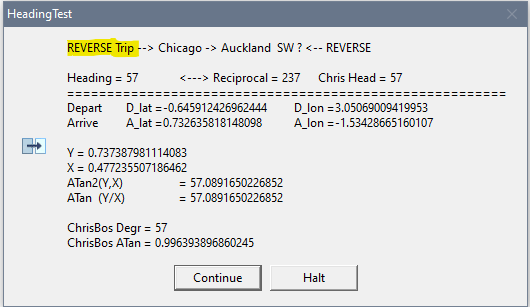# Bearing (Heading) between Two Point Calculation in CW needs ATAN2() function

I’m trying to calculate a bearing/heading between two geographical points for which I have LAT/LON in degrees like 49.12323421 -72.214342

All formulas I’ve found use ATAN2 which CW does not have.

Does anyone have a (one line) formula for this calculation, result should be in degress (0-359).

Thanks

Bostjan

fastmath.txt (1,9 КБ)
Rename attached txt to fastmath.a, then

  PRAGMA('compile(fastmath.a)')

MAP
MODULE('FastMath.a')
_Sin (REAL),REAL,NAME('__Sin')
_Cos (REAL),REAL,NAME('__Cos')
_SinCos (REAL rad, *REAL sine, *REAL cosine),PASCAL,NAME('__SinCos')
_ATan2 (REAL y, REAL x),REAL,NAME('__ATan2')
_Sqrt (REAL),REAL,NAME('__Sqrt')
END
END

1 Like

Works!

Thanks, Mike, I really apreciate it!

Looking at Wikipedia ATAN2() appears to be based on normal Arc Tangent Clarion ATAN() with some IFs for certain problem conditions of X and Y. So you could write your own.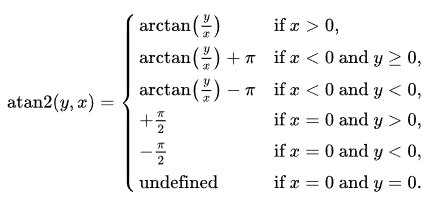Based on above reordered, untested and I don’t remember much trig.

Edit: Put the lines in order of the above 1,2,3,4,5,6. Plus the most common comes first with X>0.

!Note: Argument order is (Y,X) to match the calculation ATAN(Y/X) but is opposite conventional (X,Y) point format .
!      (Y,X) order was done in Fortran so existing ATan(Y/X) can easily change to ATan2(Y , X)
!      Some ATan2() use opposite (X,Y) like Excel and Mathematica

ATan2 PROCEDURE(REAL Y, REAL X)!,REAL
PI  EQUATE(3.1415926535898)
AT2 REAL
CODE
IF X<>0 THEN
AT2=ATAN(Y/X)                     !#1 X>0
IF    X<0 AND Y>=0 THEN AT2 += PI !#2
ELSIF X<0 AND Y<0  THEN AT2 -= PI !#3
END
ELSE !X=0 AND
IF    Y>0 THEN AT2=PI/2 * 1       !#4
ELSIF Y<0 THEN AT2=PI/2 * -1      !#5
END ! Y=0  so  AT2=0  (default)   !#6 Undefined
END
RETURN AT2


Original Post in kind of Star Wars order 6,5,4 then 1,2,3

ATan2 PROCEDURE(REAL Y, REAL X)!,REAL
PI  EQUATE(3.1415926535898)
AT2 REAL
CODE
IF    X=0 AND Y=0 THEN AT2=0          !#6 Undefined
ELSIF X=0 AND Y<0 THEN AT2=PI/2 * -1  !#5
ELSIF X=0 AND Y>0 THEN AT2=PI/2 * 1   !#4
ELSE !IF X>0 but also <0 then adjusted below
AT2=ATAN(Y/X)                     !#1 X>0
IF    X<0 AND Y>=0 THEN AT2 += PI !#2
ELSIF X<0 AND Y<0  THEN AT2 -= PI !#3
END
END
RETURN AT2

! Rad2Deg   EQUATE(57.295779513082)  !Number of Degrees in a Radian = 180/PI


ATAN() returns Radians. Multiply AT2 * Rad2Deg for Degrees. … Again not an engineer, but on the Math team … 40+ years ago.

4 Likes

@Bostjan_Laba reported to me he tested the below Clarion code ATAN2() function and it matched the FastMath _Atan2 posted by Mike.

ATan2 PROCEDURE(REAL Y, REAL X)!,REAL
PI  EQUATE(3.1415926535898)
AT2 REAL
CODE
IF X<>0 THEN
AT2=ATAN(Y/X)                     !#1 X>0
IF    X<0 AND Y>=0 THEN AT2 += PI !#2
ELSIF X<0 AND Y<0  THEN AT2 -= PI !#3
END
ELSE !X=0 AND
IF    Y>0 THEN AT2=PI/2 * 1       !#4
ELSIF Y<0 THEN AT2=PI/2 * -1      !#5
END ! Y=0  so  AT2=0  (default)   !#6 Undefined
END
RETURN AT2


First he tested it on 100 of his real world data. Next he tested it on a range of most possible values including the special cases where X and Y were =0 or <0 with code like:

  LOOP LatX=-180 TO 180
LOOP LonY=-90 TO 90  !Not sure of these
AT2Clw  = Clw_ATan2(LonY,LatX)
AT2Fast = FastATan2(LonY,LatX)
IF ABS(At2Clw - At2Fast) > 0.0001 THEN ....

2 Likes

Thanks, Carl, for posting this instead of me, I got busy in past few days.

Anyway, in addition, here’s the routine from my app directly, which does the calculation of bearing from point A (Departure) to point B (Arrival) in degrees (0-359). A and B lan/lon are in degrees, like from google maps or similar.

DATA


D_lat real !departure point latitude
D_lon real !departure point longitute
A_lat real !arrival point latitude
A_lon real !arrival poing longitude

CODE

AQ:Airport=PQ:departure
get(AirportsQ,AQ:Airport)
D_lat=AQ:Latitude
D_lon=AQ:Longitude

AQ:Airport=PQ:arrival
get(AirportsQ,AQ:Airport)
A_lat=AQ:Latitude
A_lon=AQ:Longitude

if D_lat and D_lon and A_lat and A_lon !we got data
D_lat = d_lat * 3.1415926535898/180
D_lon = d_lon * 3.1415926535898/180
A_lat = a_lat * 3.1415926535898/180
A_lon = a_lon * 3.1415926535898/180
! y= cos(A_lat) * sin(A_lon - D_lon)
! x= cos(D_lat) * sin(A_lat) - sin(D_lat) * cos(A_lat) * cos(A_lon - D_lon)
! ATAN2 = (y, x)
PQ:heading = ATAN2(SIN(A_lon - D_lon) * COS(A_lat), COS(D_lat) * SIN(A_lat) - SIN(D_lat) * COS(A_lat) * COS(A_lon - D_lon)) * 180 / 3.1415926535898
ELSE
PQ:heading = 999 !we have no data so we return "invalid" heading
END


Carl’s code for Atan2 certainly works. However, if return of positive angles is required, I have found that the following, shorter code does the job.

            Atan2 = ATAN(Y/X)
If X < 0
Atan2 += pi
ElsIf Y < 0
Atan2 += 2*pi
End


If Y/X is negative, ATAN will return a negative angle in the 4th quadrant. If X is negative, the resulting angle will be in the 2nd or 3rd quadrant. If in the third quadrant, ATAN will be positive and the result will be in the first quadrant. Therefore, ADD pi (180 degrees) for negative values of X. With a positive X but negative Y, the angle will be in the 4th quadrant and a Negative angle will be returned. Add 2*pi (360 degrees) to make it positive.
It seems in my testing that Clarion’s ATAN function returns pi/2 (90 degrees) if X is zero, anticipating the problem of division by zero. Thus, if X is zero and Y is negative add pi to the pi/2 result.

@Bostjan_Laba
You converted degrees to radians for input to the trig functions, but remember that ATAN2 if predicated on ATAN will return radians and require conversion to degrees (180/pi.)

I think that’s done wayyyyy out on the right side you’ll see * 180 / 3.1415926535898

PQ:heading = ATAN2(SIN(A_lon - D_lon) * COS(A_lat), COS(D_lat) * SIN(A_lat) - SIN(D_lat) * COS(A_lat) * COS(A_lon - D_lon)) * 180 / 3.1415926535898
! --> out here >---------------------------> keep scrolling >-----------------------------> a bit more >------------------> Look Up ^^^^^^^^^^^

!Below the wrapped line in split

PQ:heading = ATAN2( SIN(A_lon - D_lon) * COS(A_lat), |
COS(D_lat) * SIN(A_lat) - SIN(D_lat) * COS(A_lat) * COS(A_lon - D_lon) |
) * 180 / 3.1415926535898     !<--- 180/Pi



@Bostjan_Laba,
Your formulae suggest spherical trigonometry, and I assume - at least for longer distances - airlines fly great circle routes. In the case of a great circle the course changes continually along the arc.
Given that, an initial course can be calculated using usual navigational spherical trigonometry equations without using a tangent function: (here ‘t’ represents ArrivalLongitude minus DestinationLongitude.)
Hc = ASin[Sin(LatDest)∙Sin(LatDep) +
Cos(LatDest)∙Cos(LatDep)∙Cos(t)]
Distance[nm] = (90° − Hc) ∙ 60[nm/deg]
Z = ACos[(SinLatDest – SinLatDep ∙ SinHc) / (CosLatDep ∙ CosHc)]

  If Destination is East of Departure,  Initial Course = Z
If Destination is West of Departure, Initial Course = 360 – Z


Zero Degrees is a valid Latitude and valid Longitude. Either of those Zeros and your IF And And And fails so no heading is calc.

The point (0,0) is in the Atlantic Ocean so no Airport there, but a possible waypoint destination. Maybe change your AND * 3 to check OR * 3 so there must be at least 1 non-zero number. Or test INRANGE(Lats,-90,90) and INRANGE( Lons, -180,180) for valid values. Or test if your GET( Airport ) errors.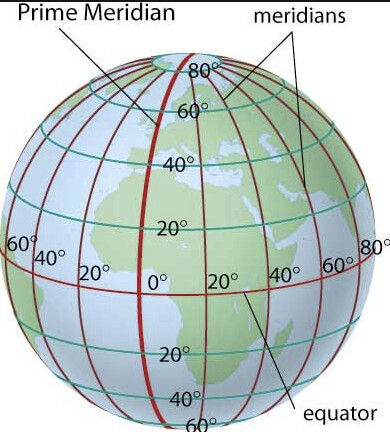1 Like

The Earth is not flat and therefore the planar Euclidian geometry is not valid on its surface if distances are large enough. Particularly, the sum of triangle angles on the sphere (on the ellipsoid for the Earth) is more than 180 degrees. The distances between two points are greater than follows from the planar geometry, and if to take altitudes into account they are even greater.

I think you’re talking about the Bearing Calculation is for a flat Earth. Per one web source:

The formula gives the initial heading for a Great-Circle Route from point A to point B. The heading will change in the course of the trip.

I tested the formula for Chicago to Auckland and it gives an Initial Heading of 244 degrees so -180 the Reciprocal Heading should be 64 degrees, but if you run the points in reverse for Auckland to Chicago the Heading is 57 which a Reciprocal of 237.

Using GcMap.com to check the calcs shows 244.6 ORD to AKL, and 57.2 AKL to ORD. It says “Initial Heading” in over the column.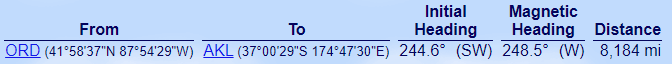Reverse trip GcMap AKL to Chicago is 57.2 not close to 64.6 the Reciprocal of 244.6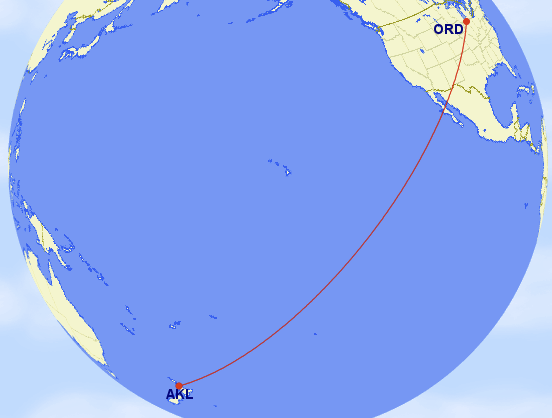What was confusing initially was Chicago to the North Pole is obviously head North on a 000 heading. But the Pole to Chicago calculation returned 268 degrees not 180 degrees. … Because everywhere is South … standing on the Pole you have to turn to 268 degrees to find your initial heading. You’re not going to navigate that with a magnetic compass, only GPS.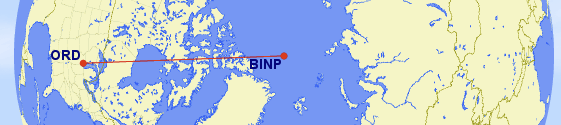You can put in 2 trips into GCMap with a comma between. That makes it easy to compare the reverse trip in one plot. The below numbers are for 2 trips: BINP-ORD,ORD-BINP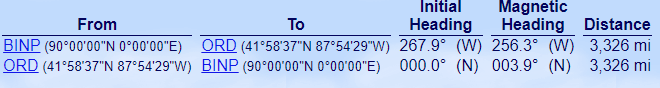If a house has Windows that all face South, and you look the Window and see a Bear … What color is the Bear? … It has to be White.

35 years ago I wrote a program to process data obtained by the hydrographic expedition: buoys with equipment which were distributed over a large area of the sea periodically registered different parameters of the water and my program processed all these data to determine how these parameters were changing in dynamics and how values in one point were dependent from values in other points. Even if distance between buoys was ~50 km, ignoring of the real form of the Earth was giving sufficient errors.

I don’t know, probably routes between airports are not so sensitive to difference between geometry on the plane and on the sphere but unlikely this difference can be ignored.

[quote=“ChrisBos, post:7, topic:6589”]
Carl’s code for Atan2 certainly works. However, if return of positive angles is required, I have found that the following, shorter code does the job.

Atan2 = ATAN(Y/X)
If X < 0 Then Atan2 += pi  ElsIf Y < 0 Then Atan2 += 2*pi .


Chris I found your code matches in my tests below of a bunch of airports.

I tried to have a mix the possible Positive and Negatives. All are done twice flipping the Departure and Arrival location so that tests more. I also include odd ones like Null Island (0,0) and the Poles.

HeadingTests PROCEDURE()
CODE    !Data from www.airnav.com or www.gcmap.com or Wikipedia
SYSTEM{PROP:MsgModeDefault}=MSGMODE:CANCOPY
!Mix  +- +-   Depart Lat  Long         Arrive Lat  Long
HeadingTest2x(41.9769403,-87.9081497 ,  49.009722,  2.547778  , 'Chicago -> Paris  NE ?')
HeadingTest2x(41.9769403,-87.9081497 , -22.81    ,-43.250556  , 'Chicago -> Rio de Janeiro  SE ?')
HeadingTest2x(41.9769403,-87.9081497 , -37.008056, 174.791667 , 'Chicago -> Auckland  SW ?')
HeadingTest2x(41.9769403,-87.9081497 , 25.7953611,-80.2901158  ,'Chicago -> Miami SE ?')
HeadingTest2x(41.9769403,-87.9081497 , 39.8616667,-104.6731667 ,'Chicago -> Denver  West?')

HeadingTest2x(-22.81    ,-43.250556  , -37.008056, 174.791667 , 'Rio de Janeiro -> Auckland  West ?')
HeadingTest2x(-22.81    ,-43.250556  ,  49.009722,   2.547778 , 'Rio de Janeiro -> Paris  NE ?')   !GIG-CDG  - - + +
HeadingTest2x(41.275278 , 28.751944 ,  49.009722,    2.547778 , 'Istanbul -> Paris  NW ?')         !IST-CDG  + + + +

HeadingTest2x(41.9769403,-87.9081497 , 000       , 0000       , 'Chicago-Null Island Africa (0,0) E?')
HeadingTest2x(41.9769403,-87.9081497 , 90        , 0          , 'Chicago-North Pole  N?')
HeadingTest2x(90        , 0          , -90       , 0          , 'North to South Pole S?')
RETURN


This calls, then again with flipped Arrive->Depart to test the REVERSE. Usually the Initial Heading is not the same as the reciprocal of the original Deoart → Arrive

HeadingTest2x PROCEDURE(REAL D_lat , REAL D_lon , REAL A_lat , REAL A_lon , STRING TripDesc)
CODE
HeadingTest1(a_lat ,a_lon, d_lat,d_lon ,'REVERSE Trip --> '& CLIP(TripDesc) &' <<-- REVERSE')
RETURN


This is Bostjan’s code as unmodified as possible. I split out X and Y as variables. I changed the IF validation to INRANGE. I added Chris’ calc to compare and it always matched … to my eye … I should have had code to check.

HeadingTest1 PROCEDURE(REAL D_lat , REAL D_lon , REAL A_lat , REAL A_lon , STRING TripDesc)
Y   REAL
X   REAL
Pi314   EQUATE(3.1415926535898)
ChrisATan REAL
ChrisDeg  REAL
CODE
IF  INRANGE(D_lat,  -90,  90) |  !was IF D_lat And D_Lon but that failed for (0,0)
And INRANGE(D_lon, -180, 180) |
And INRANGE(A_lat,  -90,  90) |
And INRANGE(A_lon, -180, 180) THEN  !we got data
D_lat = d_lat * 3.1415926535898/180
D_lon = d_lon * 3.1415926535898/180
A_lat = a_lat * 3.1415926535898/180
A_lon = a_lon * 3.1415926535898/180
Y = SIN(A_lon - D_lon) * COS(A_lat)
X = COS(D_lat) * SIN(A_lat) - SIN(D_lat) * COS(A_lat) * COS(A_lon - D_lon)
Heading = ATAN2( Y , X ) * 180 / 3.1415926535898
ELSE
END
ChrisATan = ATAN(Y/X)   !Chris Bos posted this "if return of positive angles is required, I have found that the following, shorter code does the job"
If    X < 0 THEN ChrisATan += PI314
ElsIf Y < 0 THEN ChrisATan += 2 * PI314
END

CASE Message(TripDesc & |
'      Chris Head = '& ChrisDeg & |
'|={55}' & |
'|Depart <9> D_lat ='& D_lat & '<9> D_lon ='& D_lon & |
'|Arrive <9> A_lat ='& A_lat & '<9> A_lon ='& A_lon & |
'||Y = ' & Y &'|X = '& X & |
'|ATan2(Y,X) <9>= '& ATan2(Y,X) * Rad2Deg & |
'|ATan  (Y/X) <9>= '& ATan(Y/X) * Rad2Deg & |
'||ChrisBos Degr = '& ChrisDeg & |
'|ChrisBos ATan = '& ChrisATan & |